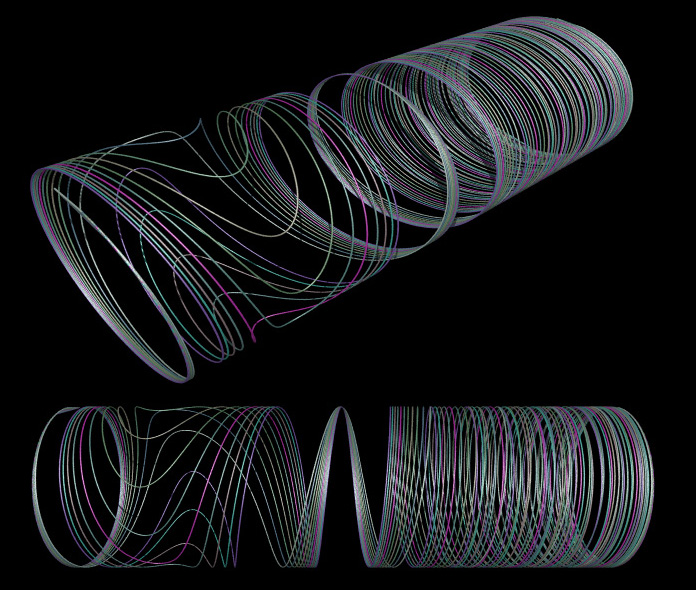# Carotid Function Fractal

Written by Paul Bourke
April 1998

So called "Carotid-Kundalini" curves of degree i are defined as

f(x) = cos(i x acos(x))

where i ranges from 1 upwards, and -1 <= x <= 1

Superimposing the Carotid-Kundalini curves up to some degree on top of each results in a seemingly self similar structure.

First 10 curves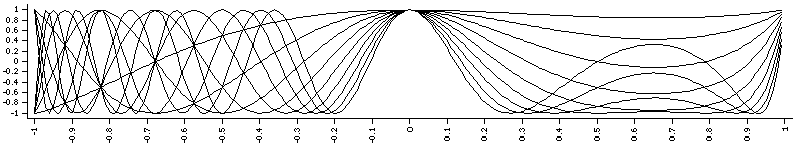First 20 curves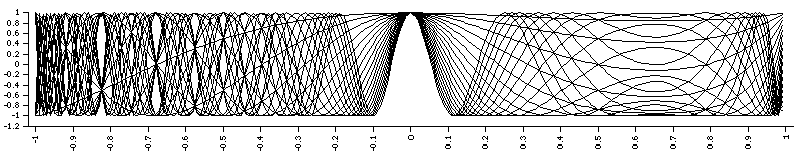First 30 curvesZooms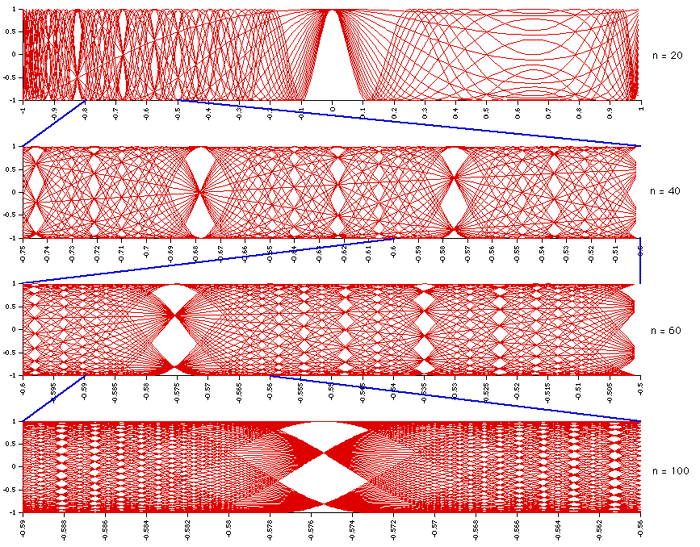C Source

```#include "stdio.h"
#include "stdio.h"
#include "math.h"

int main(int argc,char **argv)
{
int i,n;
double x,y,dx;

if (argc < 3) {
fprintf(stderr,"Usage: %s n dx\n",argv);
exit(0);
}
n = atoi(argv);
dx = atof(argv);

for (x=-1;x<=1;x+=dx) {
printf("%g ",x);
for (i=1;i<n;i++) {
y = cos(i * x * acos(x));
printf("%g ",y);
}
printf("\n");
}
}
```

Extension to 3D

The Carodit functions can be extended into 3D in a number of ways, the one used here is as follows where a and b are scaling factors.

 f(x,y,z) = (a x, b cos(i x acos(x)), b sin(i x acos(x)))

The first 10 curves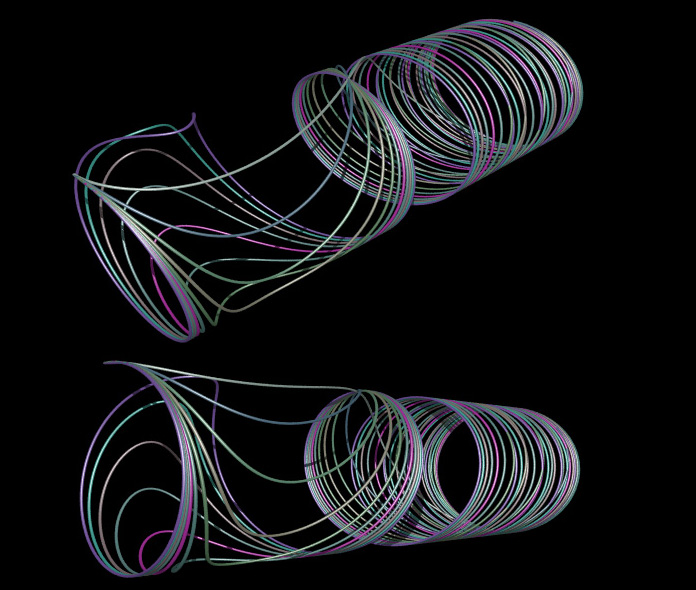The second 10 curves